# 1 | 绪论

## 1.0 | 考题

1. 人工智能定义
2. 举出5个人工智能方面的应用并简单介绍
3. 简述人工智能三大学派及其观点

## 1.1 | 人工智能三大学派

1. 1936发表论文, 提出图灵机理论
2. 1950年发表论文, 提出图灵测试

1956年达特矛斯会议「人工智能」正式诞生

# 2 | 知识表示

## 2.0 | 考题

1. 至少简述四种知识表示方法及其特点
2. 什么是本原问题

## 2.1 | 知识表示方法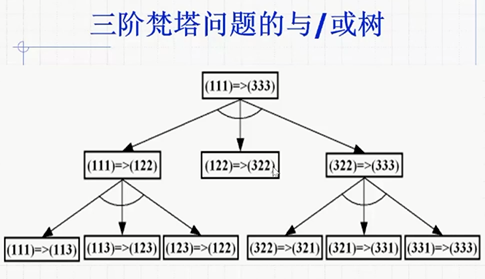# 3 | 经典逻辑推理

2道必考题:

• 归结反演的大题

• 用谓词逻辑表示下列句子

## 3.1 | 代换(置换)

$x_i$为某一公式中互不相同的变元

• 假言推理
• 拒取式
• 假言三段论

## 3.4 | 归结演绎推理

### 将谓词公式化为子句集的步骤

1. 消去$\rightarrow$

2. 将$\lnot$移动到紧靠谓词的位置上

3. 重命名变元使得不同约束变元名字不同
4. 消去$\exists$
• 若$\exists$不在$\forall$的辖域内, 用常量替换变元即$a/x$
• 若$\exists{y}$在$\forall{x}$的辖域内, 用skolem函数$f(x_1, x_2, \dots)$替换变元即$f(x)/y$
5. 消去$\forall$化为合取范式
6. 消去$\land$得到子句集

e.g.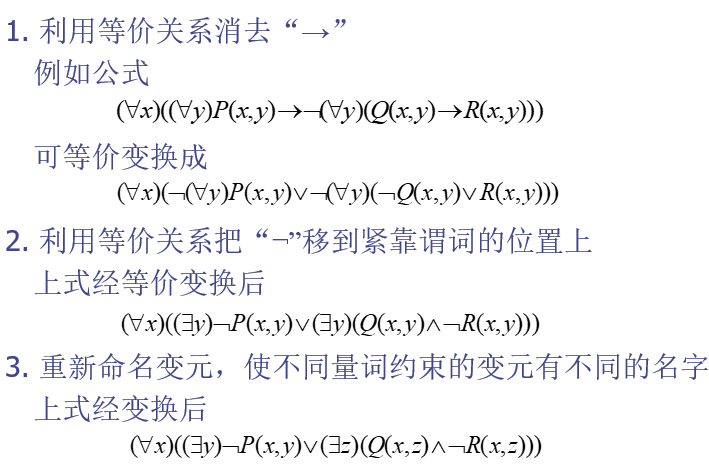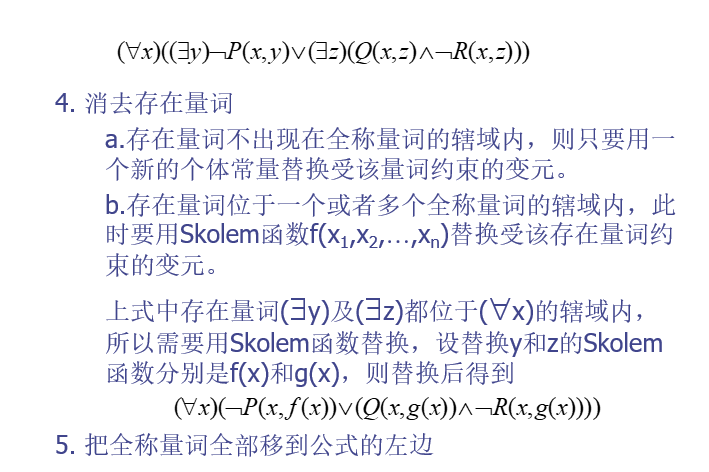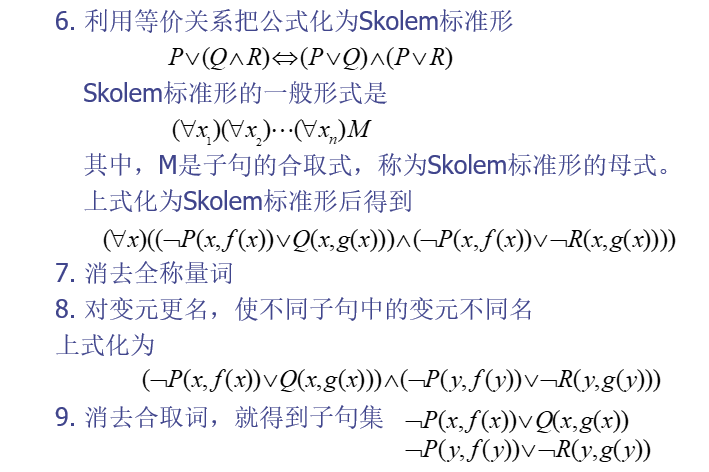### 利用归结反演的证明步骤

1. 否定Q，得到¬Q；

2. 把¬Q并入到公式集F中，得到{F, ¬Q};

3. 把公式集{F, ¬Q}化为子句集S；

4. 应用归结原理对子句集S中的子句进行归结，并把每次归结得到的归结式都并入S中。如此反复进行，若出现了空子句，则停止归结，此时就证明了Q为真。

### 利用归结原理求取问题的答案

1. 把已知前提用谓词公式表示出来，并且化为相应的子句集。设该子句集的名字为S。

3. 把此析取式化为子句集，并且把该子句集并入到子句集S中，得到子句集S’。

4. 对S’应用归结原理进行归结。

e.g.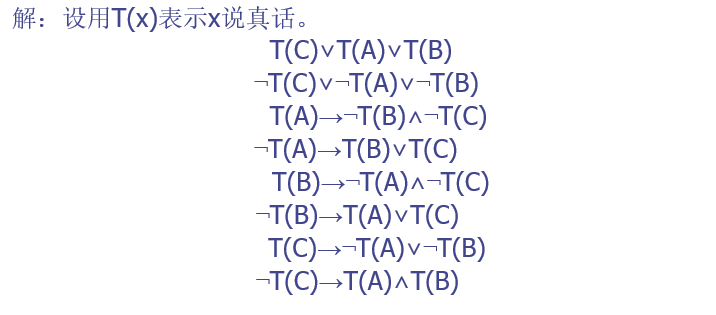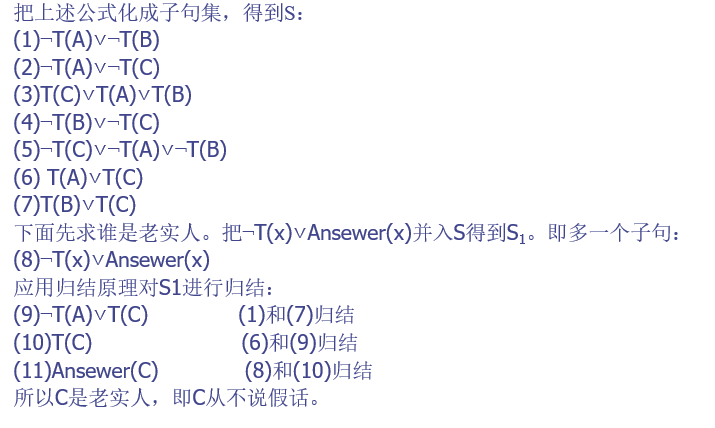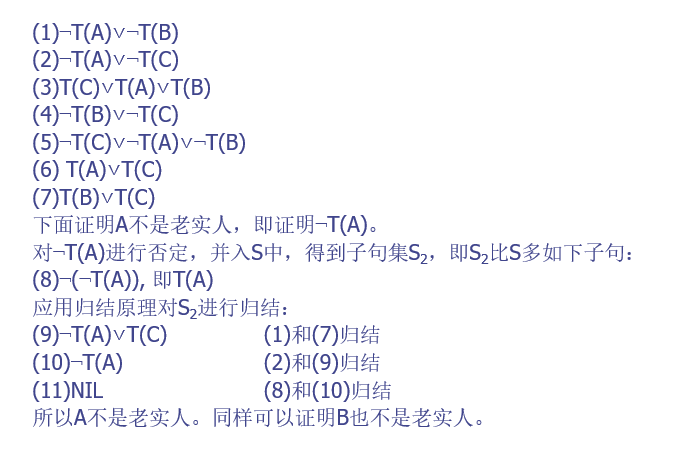# 4 | 不确定推理

## 4.0 | 考题

1. 给你几个CF让你求一个CF, 需要用到的公式:
• 组合证据不确定度算法(and取小, or取大)
• 不确定性的传递算法
• 结论不确定性的合成算法

## 4.2 | 主观贝叶斯方法

$LS$是规则的充分性度量, 定义为:

$LS$指出了$E$对$H$的支持程度

LN是规则的必要性度量, 定义为:

$LN$指出了$\lnot E$对$H$的支持程度

### 例题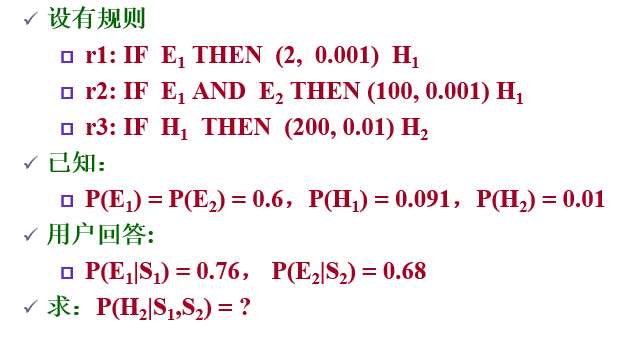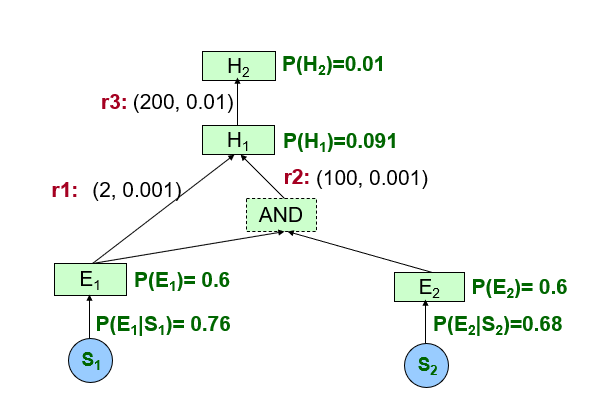1. $P(H_1|S_1)$
2. $P(H_1|(S_1 \text{AND} S_2))$
3. $P(H_1|S_1,S_2)$
4. $P(H_2|S_1,S2)$

1. EH公式即 (考试会给)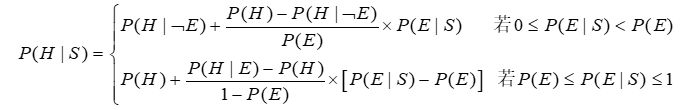2. $P(H|E)=\frac{LS \times P(H)}{(LS-1) \times P(H)+1}$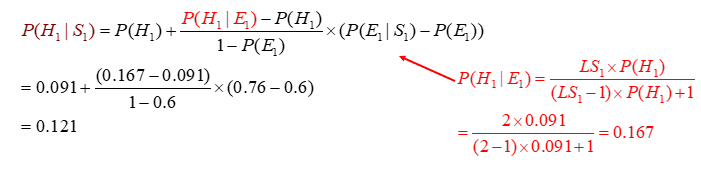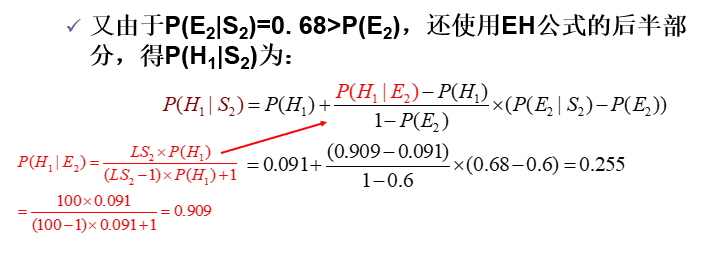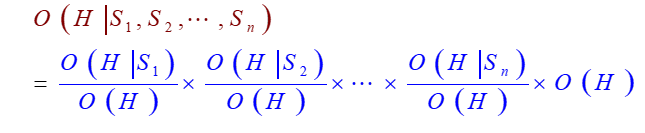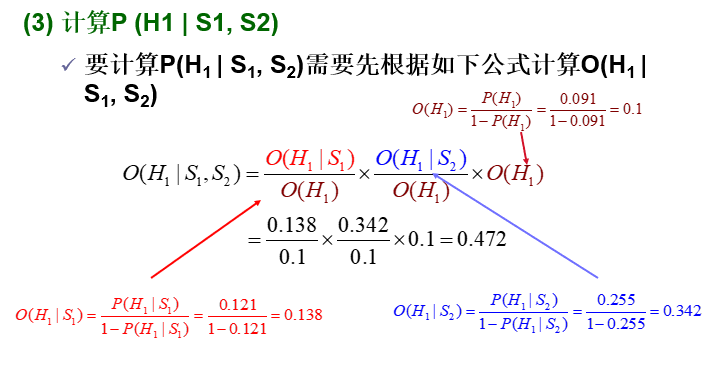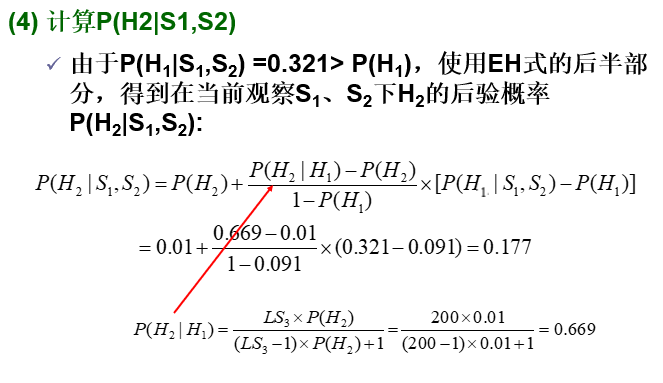## 4.3 | 可信度方法

$CF(H,E)$指该知识的可信度, 称为可信度因子/静态强度, 范围在-1~1

$CF(H,E)$定义为:

### 组合证据不确定度算法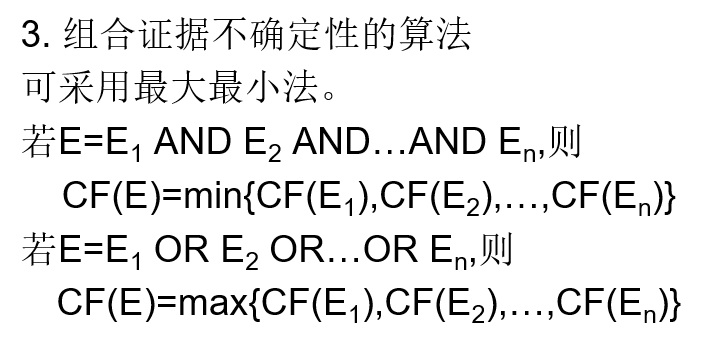### 结论不确定性的合成算法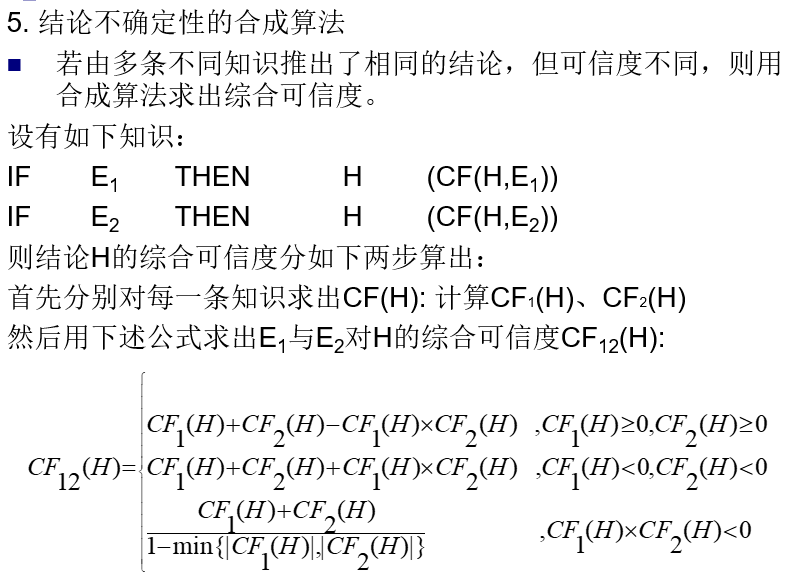e.g.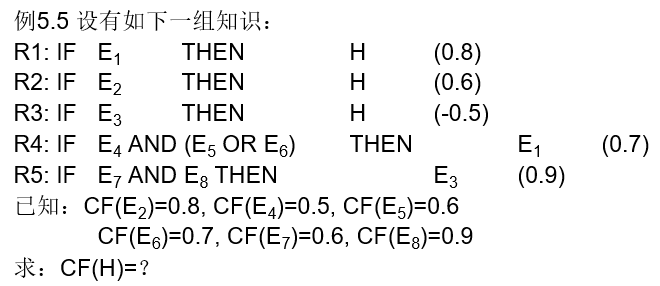## 4.4 | 带阈值和权值的可信度方法

• 带权值的知识计算组合不确定度的方法就是分别乘以权值然后加起来(注意这里w1 + w2 + … = 1)
• λ的作用就是CF(E)需要大于等于λ, 这条知识才能被运用, 如果小于λ, 就丢掉

e.g.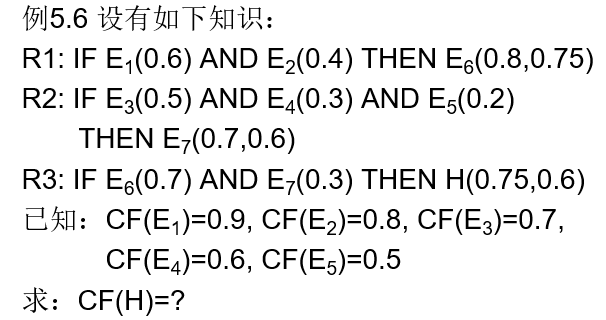# 5 | 模糊集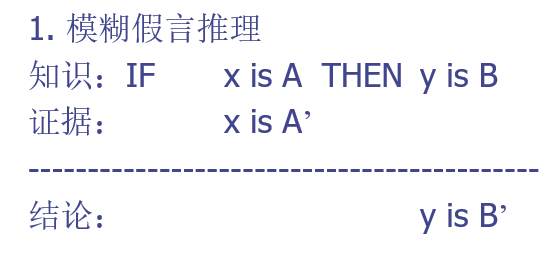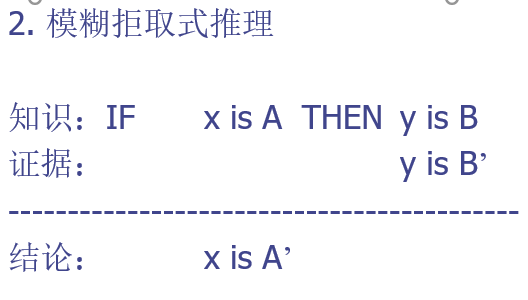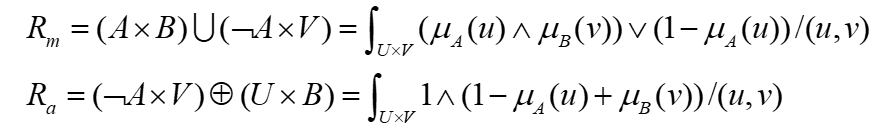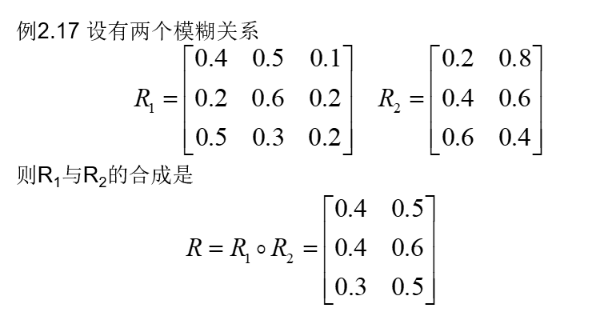e.g.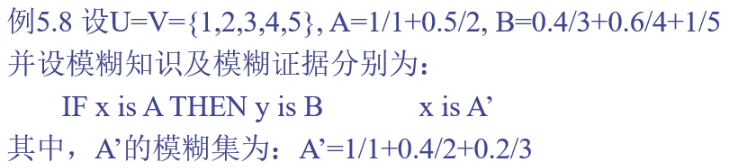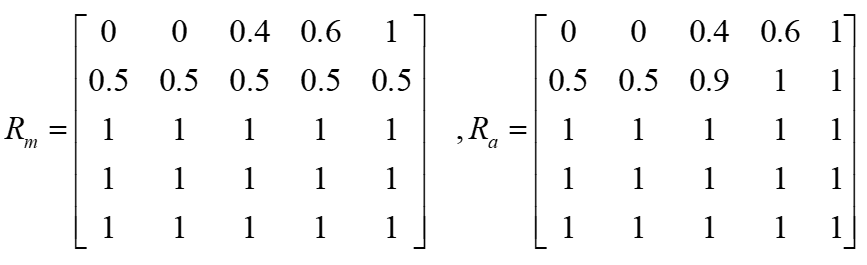# 6 | 搜索

A*的h(x)有两种策略:

• 错放的棋子数
• 所有棋子与目标位置的曼哈顿距离之和

1. 需要标出搜索状态的序号(从1开始)
2. 需要标出原状态和目标状态
3. A*的g(x)从0开始, 取值是搜索树当前层数-1, 即从初始状态到目前移动的步数

e.g.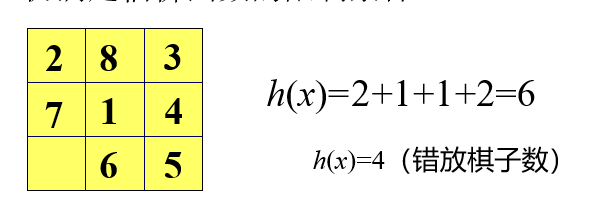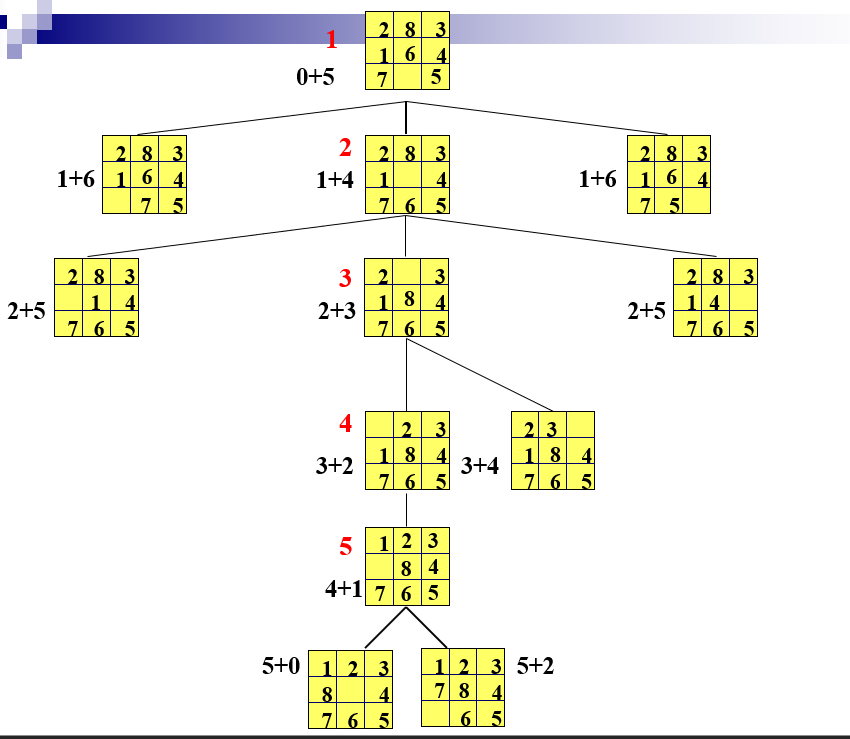BFS得搜索树如下: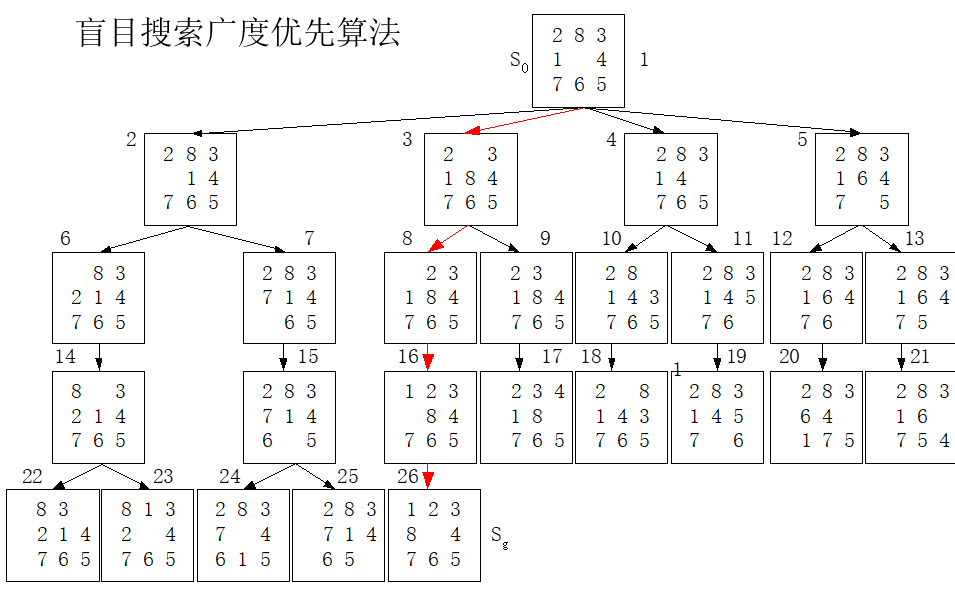DFS得搜索树如下:(老师上课说画图不能提前猜到答案直接一路DFS直接搜到了, 所以我们要伪装一下…)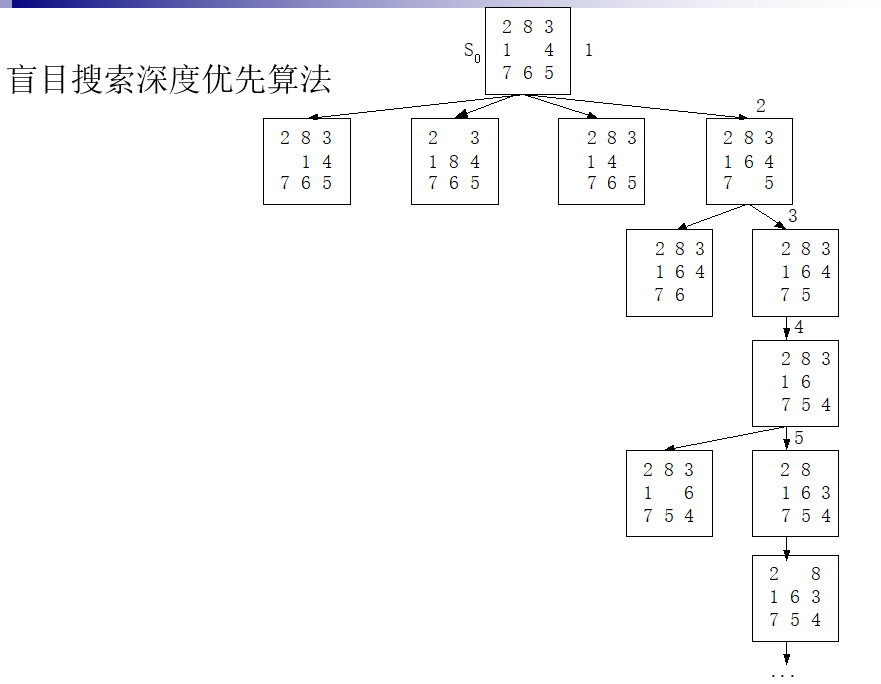# 7 | 计算智能

alphago: 神经网络系统, 蒙特卡洛算法, 深度学习

「人工智能导论」期末复习
https://blog.roccoshi.top/posts/60546/

RoccoShi

2021年5月31日